# Error in trapezoidal integration using a Programming language

nughii
Summary:: I want to iterate a mathematical model using a programming language. The equation of the mathematical model is simple. The following is a brief explanation.

I want to iterate a mathematical model using a programming language. The equation of the mathematical model is simple. The following is a brief explanation.

Looping steps 1 until 4:
 A = integral (x)
 B = 1.23456-A
 C = 1 / B
 x=C

Integration using the trapezoid method. I experience problems when the value of B approaches 0, for example B = 0.00001. Then, the e quation 3 will be of near infinite value.

How to resolve this error?

Gold Member
What is the ##1.23456## why are you doing that? How is this area? I'm not seeing it. Is ##x## initialized?

Something that might be helpful is a little diagram of what you are doing.

Okay so the integral of ##x## you're getting the area under curve ##x##. I would imagine you're done there if you are getting the area of ##x##. What is ##x##?

Then you do some seemingly arbitrary number subtracting the area? Why?

Then you get its reciprocal? What? Why?

nughii
I am sorry. This is the complete equation:

First Equation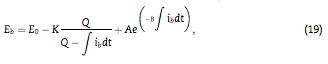##I_b## =battery current.
##E_0## =constant voltage.
##E_b## =the battery voltage.
##Q## =is the nominal capacity.
##A##, ##B##, and ##K## are battery's constant.

 Second Equation
In the second equation, I calculate the next ##Ib## by:
$$Ib=\frac{(P_{supply} - P_{demand})}{E_b}$$

 Third Equation
I calculate the ##\int I_b## by trapezoid method.

All of this equation are in a loop algorithm:
1.Calculate ##E_b##
2. Calculate ##I_b##
3. Calculate ##\int I_b##
4. Back to first step

The initial value of ##I_b## is 0.

I get the error when the value of ##E_b## is nearly 0. It will cause the value of the next ##I_b## too large.

Thank you🙏

Mentor
The battery voltage shouldn't be very close to 0 for realistic batteries. If your calculation produces numbers close to zero then something else went wrong.

nughii
The first and the second equation are from different paper. I think I can join this equation. Maybe I wrong in this part.

So, is it right if the second equation to be time-dependent too?

Last edited:
Mentor
If your current varies with time then the power or the voltage must vary.

It would be easier if you start with a description of the actual problem you want to solve.

Gold Member
Is the question more about the equation used for the battery or how the integral is being performed? From the title I thought there was an integral problem, but all that is shown is the use of some function called integral applied to ##x##. If you're concerned about how the integral function is working is there any way you can show us what is going on inside of that function or is it something builtin to the programming language you are using?

nughii
I doubt whether my error is in the integral () function or mathematical modeling error.
My teacher gave me a hint about it so that I would try to learn the computational approach in the integration process. However, I still haven't found another clue.

I wrote a program using structured text language.
Here is my integral function in pseudocode. I created this function by own.

#input of integral() function
Ib=>charge/discharge current

#parameter
dt=>iteration step

#algorithm
diff_I=(I_last+Ib)*(dt/2)
I_result=I_accum+diff_I
I_last=Ib
I_accum=I_result

#output of integral() function
I_result=>the integral of Ib

nughii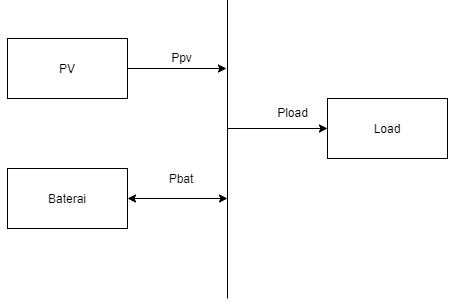A description of my problem:

I want to simulate a microgrid using 4DIAC Forte. I have 3 block functions: PV,Battery, and Load. Now, I want to combine these block functions to simulate the microgrid.

1. PV Block function
#input : temperature & solar iradiation value
# output : PV voltage, PV current, and PV power

2. Battery Block Function
#input: Charge/ discharge current
#equation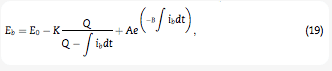#output: Battery voltage

#input:resistance

In order to combine these 3 block functions, I use this equation: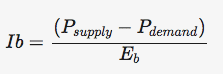##I_b##= battery charge/discharge current
##P_{supply}=P_{pv}+P_{battery}##
##E_b##=battery voltage

I have validated the PV model and the battery against the datasheet. Both are valid. But I get an error when I combine these block function using the equation above. The error occurred when ##E_b## value is close to 0.

Last edited:
Gold Member
I think it would be a good idea to have your title change. Can you see why "Error in trapezoid integration using Programming language" might attract the wrong people for your battery problem?

Something that might be helpful is explaining what each term is in that voltage ##E_b## the first equation you're using. Quite honestly I've never seen it before, but I admit I don't have experience with batteries.

For example first term ##E_0## okay initial voltage. Second term... something scaling to charge? I'm guessing ##K## is in the units of voltage since the fraction is charge over charge (looks like a reciprocal of percent charge)? Third term I have no idea. Some constant times an exponential constant times charge? What are all of these? Power equation looks acceptable, but I'm not sure if it's acceptable to use it for your first equation isn't that just instantaneous current not sure if plugging that into equation as an integral makes sense-- I'm not claiming it's wrong, but I'm just wondering if it makes sense (I don't know).

Where do you think things went wrong. The equation? Units? Assumptions? It's not clear to me what needs help just not getting expected output based on possibly incomplete information or copied formulas?

As for the programming I've seen some interesting stuff when near critical numbers like zero can cause convergence issues. Kind of reminds me of another thread speaking of the maths and programming- might be interesting for thought even though it's not a battery.

Mentor
Are you iterating everything over time? So your whole "integral" is just a single trapezoidal time step? Why don't you write ##I_b~\Delta t## then? I'm not sure how an integration scheme would use a trapezoid but that's a question for later.

What determines the load? Is that some external input?

I still think that Eb can never get close to zero. Not for realistic systems at least. It's no surprise that you get strange results from the current exploding. Smaller time steps would change that but they don't change the underlying problem that leads to the unphysical Eb.

Mentor
I'm not sure how an integration scheme would use a trapezoid but that's a question for later.
Trapezoidal integration is a numerical technique for approximating an integral by summing the areas of several trapezoids. Each subinterval, of width ##\Delta t##, determines a trapezoidal region whose parallel sides are ##f(t_i)## and ##f(t_i + \Delta t)##.

Mentor
Yes I know what trapezoidal integration is (...), but it's a method to calculate an integral for a function you know in advance. An integration scheme for differential equations has to calculate these function values "on the fly", which needs different methods.

nughii
Are you iterating everything over time? So your whole "integral" is just a single trapezoidal time step? Why don't you write ##I_b~\Delta t## then? I'm not sure how an integration scheme would use a trapezoid but that's a question for later.

I am doing integrals, from 0 to ##t_{finish}##. Each step increases 0.0001 seconds.

What determines the load? Is that some external input?

I use an external input for the load. For example ##P = 10W## for ##0<t<=5 seconds## and ##P = 5W## for ##6<t<10## seconds.

#####################################

I use the battery model from this paper : https://www.researchgate.net/publication/304745172_Efficient_simulation_of_Hybrid_Renewable_Energy_Systems

The PV model from this paper:
https://cv4.ucm.es/moodle/pluginfil...rce/content/23/PFM_Raul_Rodriguez_Pearson.pdf

The equation for calculate ##I_{charge/discharge}## : https://www.researchgate.net/publication/271615847_Comparative_evaluation_of_different_power_management_strategies_of_a_stand-alone_PVWindPEMFC_hybrid_power_system

This is the example of my simulation result.
The x-axis is time. The left-y-axis is power. The right-y-axis is battery's SoC.

There are significant errors in ##0<t<2 seconds##.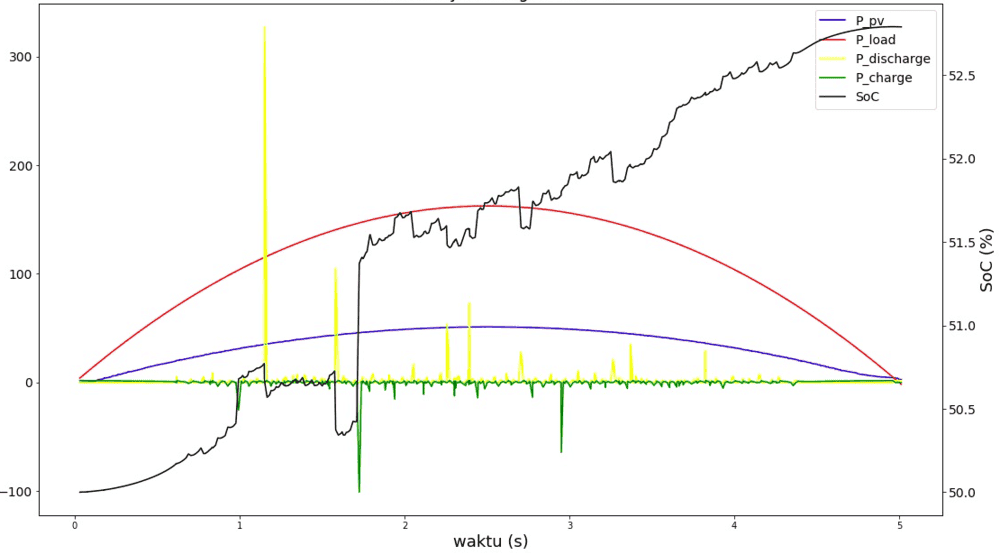Mentor
How can your battery charge up if the load needs more power than the solar cell provides? How can P_charge jump so erratically when the inputs don't change? There are some odd features here.

nughii
How can your battery charge up if the load needs more power than the solar cell provides? How can P_charge jump so erratically when the inputs don't change? There are some odd features here.
Yes, there is something wrong, but I am still looking for a solution.

nughii
May I ask for advice, where should I start to fix this? Thank you

Gold Member
Some general approaches for debugging:
• Simplify by eliminating some stuff
• Try without a load and a fixed value for P_pv
• Does the battery stop charging at the correct Voltage? State-of-Charge (SoC)?
If not, the model of the PV panel and/or the battery is/are wrong.
• Try P_pv=0, battery SoC=100% and fixed load (Battery SoC should drop faster as time progresses, especially the first and last 5%)
• Does the load stop drawing power when battery SoC is approaching dead? Does SoC ever go negative?
If not, the model of the battery and/or the load is/are wrong.
• Pick some variables that can be fixed and make them a constant for a run
• P_pv and P_load would be good candidates to be steady and equal to each other
• Run them and see if the results are reasonable
• If not, print the internal variables in the internal loop(s) before, during, and after the time that gives bad results. (Hard copy to a printer is MUCH easier to cross-check with. You can lay many sheets out on a desk, the floor, or a wall and easily do multi-way comparisons. Be sure that each sheet notes any program changes and any fixed/initial values of variables. Otherwise you lose track after about 3 iterations!)

A few of notes:
• Constant power loads are not very common. Most have a resistive component such that power is directly proportional to Voltage.
• This includes batteries which over much of their range have a super-linear decrease in output voltage with SoC,
• The integration step of 10-4 over 10 sec. is a prime suspect for problems. You may find that with the internal representation of numbers in the computer, that adding a small value to a large value does not yield the expected result.
• A possible work-around is to increase the time step, perhaps to 10-2 or 10-1 sec. If you must handle fast transients, then calculate an average value within each integration step with the needed resolution.

Well, that should keep you busy for awhile... and it's late, I'm falling asleep.😴

Cheers,
Tom

••mfb, sysprog and nughii
nughii
Thank you very much for the advice 🙏

•Tom.G